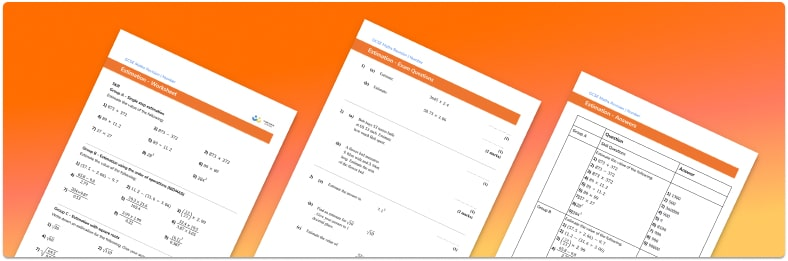# Estimation Worksheets• Section 1 of the estimation worksheet contains 27 skills based index notation, squares and cubes questions, in 3 groups to support differentiation
• Section 2 contains 3 applied index notation, squares and cubes with a mix of worded problems and deeper problem solving questions
• Section 3 contains 3 foundation and higher level GCSE exam style index notation, squares and cubes questions
• Answers and a mark scheme for all 33 questions
• Follows variation theory with plenty of opportunities for students to work independently at their own level
• All questions created by fully qualified expert secondary maths teachers
• Suitable for GCSE maths revision for AQA, OCR and Edexcel exam boards

• This field is for validation purposes and should be left unchanged.

You can unsubscribe at any time (each email we send will contain an easy way to unsubscribe). To find out more about how we use your data, see our privacy policy.

### Estimation at a glance

Estimating involves rounding numbers to make calculations easier; we use estimation to give an approximate answer to a calculation. The estimated answer will not be exactly the same as the correct answer but it will be similar and the place value of the number is likely to be the same.

When estimating in maths, we usually round numbers to 1 significant figure. For example, a 3 digit number would be rounded to the nearest hundred. We can estimate sums, differences, products and quotients and can apply estimation to multistep problems. We can also use estimation to find approximate answers to square root questions.

Estimating is very commonly used in real life, for example estimating the numbers of items we can buy at the shop.

Looking forward, students can then progress to additional number worksheets, for example aorFor more teaching and learning support on Number our GCSE maths lessons provide step by step support for all GCSE maths concepts.

## Do you have KS4 students who need more focused attention to succeed at GCSE?There will be students in your class who require individual attention to help them succeed in their maths GCSEs. In a class of 30, it’s not always easy to provide.

Help your students feel confident with exam-style questions and the strategies they’ll need to answer them correctly with our dedicated GCSE maths revision programme.

Lessons are selected to provide support where each student needs it most, and specially-trained GCSE maths tutors adapt the pitch and pace of each lesson. This ensures a personalised revision programme that raises grades and boosts confidence.

Find out more